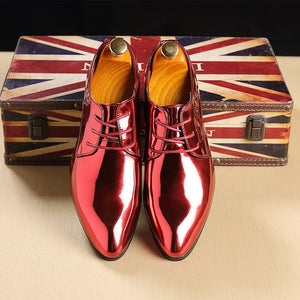# Trend Pointed Toe Casual Hard-wearing Shoes

• \$60.57
Unit price per
Shipping calculated at checkout.

Upper Material: Synthetic

Toe Shape: Pointed Toe

Outsole Material: Rubber

Insole Material: PU

Lining Material: PU

Shoes Type: Derby Shoes

Closure Type: Lace-Up

Season: Spring/Autumn

Fit: Fits true to size, take your normal size

Pattern Type: Solid

1,Note Our size is not standard US size, and it is only equal to foot length!

US5.5 =EU37=235mm=9.25in(bare feet length)

US6.0 =EU38=240mm=9.45in

US6.5 =EU39=245mm=9.65in

US7.0 =EU40=250mm=9.84in

US8.0 =EU41=255mm=10.04in

US8.5 =EU42=260mm=10.24in

US9.5 =EU43=265mm=10.43in

US10.0=EU44=270mm=10.63in

US11.0=EU45=275mm=10.83in

US12.0=EU46=280mm=11.02in

US12.5=EU47=285mm=11.22in

US13.0=EU48=290mm=11.42in